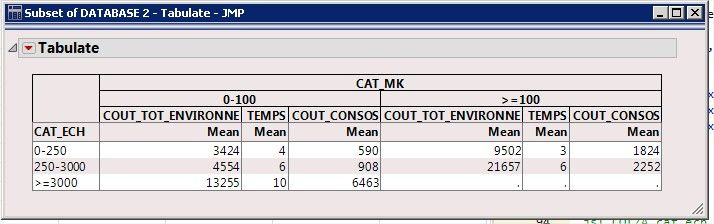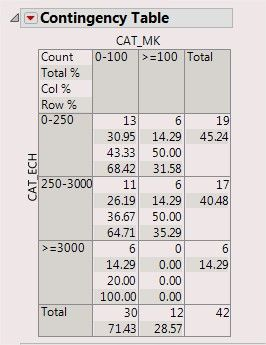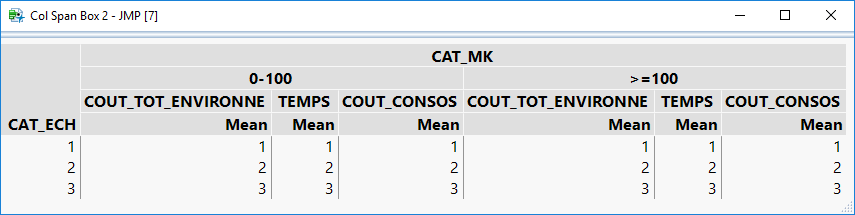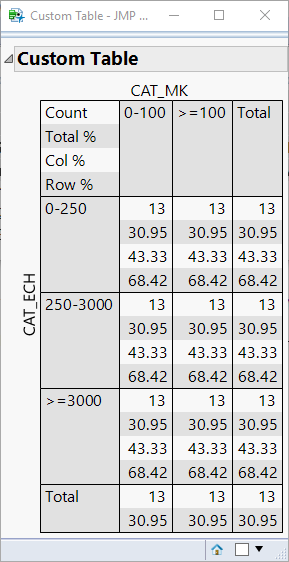Choose Language Hide Translation Bar
Highlighted

## Create a tab box with a cross tab box design

Hi all,

Not sure this is possible, but I try my luck.

I would like to create (in jsl) kind of a cross tab box, but customized with my own values. To make myself better understood:

Here is a tabulate (FIG. 1) containing the data I want to display:FIG. 1 - Tabulate

This is fine, but not quite what I want. What I would like to obtain is something similar to the cross tab box we get when calling a contingency analysis, such as in FIG.2:FIG.2 - contingency table

BUT instead of "Count", "Total%" and so on, I would put into the cells the mean values that correspond to my analysis columns in tabulate.

The features I like in this design and which I don't have in the tabulate version are :

- the possibility to display several values in one cell (and display legend in the top left hand corner)

- the absence of the "mean" line under the column headers

I couldn't find a way of converting my tabulate into what I want using tab box. Do you think it would be possible?

Thanks

1 ACCEPTED SOLUTION

Accepted Solutions
Highlighted

## Re: Create a tab box with a cross tab box design

You can fairly easily recreate the Tabulate using a TableBox and ColSpan Boxes. See below for an example.

``````Names Default To Here( 1 );
New Window( "Col Span Box",
Table Box(
Number Col Box( "CAT_ECH", {1, 2, 3} ),
Col Span Box(
"CAT_MK",
Col Span Box(
"0-100",
Col Span Box("COUT_TOT_ENVIRONNE",Number Col Box( "Mean", {1, 2, 3} )),
Col Span Box("TEMPS",Number Col Box( "Mean", {1, 2, 3} )),
Col Span Box("COUT_CONSOS",Number Col Box( "Mean", {1, 2, 3} ))
),
Col Span Box(
">=100",
Col Span Box("COUT_TOT_ENVIRONNE",Number Col Box( "Mean", {1, 2, 3} )),
Col Span Box("TEMPS",Number Col Box( "Mean", {1, 2, 3} )),
Col Span Box("COUT_CONSOS",Number Col Box( "Mean", {1, 2, 3} ))
)
)
)
);``````

Here is what the Col Span Box method looks like:Since the CrossTabBox DisplayBox is not scriptable using JSL, you would need to use a different method to recreate something like what is used in the Contingency platform.

You can do this using a concept I have been working on to use a LineupBox to create your own custom tables. This method uses a custom function for the creation of each cell to avoid a lot of code duplication. See my comments for details.

``````
//helper function to create cells in the lineupBOx "table"
getCell = Function( {values, altShading, justify = "left"},
{Default Local},

// Create a lineup box to add each of the values to
lb = Lineup Box( N Col( 1 ), SPacing( 0 ) ); // 1 column and no spacing

// loop through and add each value
For( i = 1, i <= N Items( values ), i++,

// if justify is left, put the spacer box on the right, otherwise, put it on the left
If( justify == "left",
// borderBox to give values some spacing (and for shading)
b = Border Box( Right( 5 ), Left( 5 ), top( 1 ), bottom( 1 ),
H List Box(
//Text box with current value
Text Box( values[i] ),
// Spacing to fill the cell shading all the way to the right (will grow horizontally, but not vertially)
Spacer Box( <<Set Max Size( 99999, 0 ) )
)
)
,
b = Border Box( Right( 5 ), Left( 5 ), top( 1 ), bottom( 1 ),
H List Box(
// Spacing to right align the text. the spacer will grow horizontally, but not vertially)
Spacer Box( <<Set Max Size( 99999, 0 ) ),

//Text box with current value
Text Box( values[i] )
)
)
);

// if altShading is turned on

// check if this is an even numbered row
If( Mod( i, 2 ) == 0,
//set the background color to a light-gray
b << Background color( RGB Color( 225, 225, 225 ) )
),

//when altShading is off, we set the background color to a light-gray for all values
b << Background color( RGB Color( 225, 225, 225 ) )
);

// Add the BorderBox to the LineupBox
Insert Into( lb, b );
);

// Return the LineupBox with a Border with lines around it
Return(
Border Box( Right( 0 ), Left( 0 ), top( 0 ), bottom( 0 ), sides( 15 ),
lb
)
);

);

New Window( "Custom Table",
Outline Box( "Custom Table",
Border Box( Right( 5 ), Left( 5 ), top( 5 ), bottom( 5 ),
H List Box(
V Center Box( Text Box( "CAT_ECH", <<Rotate Text( "left" ) ) ),
V List Box(
H Center Box( Text Box( "CAT_MK" ) ),
Lineup Box( N Col( 4 ), spacing( 0 ),

//Row 1
getCell( {"Count", "Total %", "Col %", "Row %"}, 1 ),
getCell( {"0-100", "", "", ""}, 0 ),
getCell( {">=100", "", "", ""}, 0 ),
getCell( {"Total", "", "", ""}, 0 ),

//Row 2
getCell( {"0-250", "", "", ""}, 0 ),
getCell( {"13", "30.95", "43.33", "68.42"}, 1, "Right" ),
getCell( {"13", "30.95", "43.33", "68.42"}, 1, "Right" ),
getCell( {"13", "30.95", "43.33", "68.42"}, 1, "Right" ),

//Row 3
getCell( {"250-3000", "", "", ""}, 0 ),
getCell( {"13", "30.95", "43.33", "68.42"}, 1, "Right" ),
getCell( {"13", "30.95", "43.33", "68.42"}, 1, "Right" ),
getCell( {"13", "30.95", "43.33", "68.42"}, 1, "Right" ),

//Row 4
getCell( {">=3000", "", "", ""}, 0 ),
getCell( {"13", "30.95", "43.33", "68.42"}, 1, "Right" ),
getCell( {"13", "30.95", "43.33", "68.42"}, 1, "Right" ),
getCell( {"13", "30.95", "43.33", "68.42"}, 1, "Right" ),

//Row 5
getCell( {"Total", ""}, 0 ),
getCell( {"13", "30.95"}, 1, "Right" ),
getCell( {"13", "30.95"}, 1, "Right" ),
getCell( {"13", "30.95"}, 1, "Right" )
)
)
)
)
)
);``````

Here is what the resulting window will look like with this method:Justin
4 REPLIES 4
Highlighted

## Re: Create a tab box with a cross tab box design

You can fairly easily recreate the Tabulate using a TableBox and ColSpan Boxes. See below for an example.

``````Names Default To Here( 1 );
New Window( "Col Span Box",
Table Box(
Number Col Box( "CAT_ECH", {1, 2, 3} ),
Col Span Box(
"CAT_MK",
Col Span Box(
"0-100",
Col Span Box("COUT_TOT_ENVIRONNE",Number Col Box( "Mean", {1, 2, 3} )),
Col Span Box("TEMPS",Number Col Box( "Mean", {1, 2, 3} )),
Col Span Box("COUT_CONSOS",Number Col Box( "Mean", {1, 2, 3} ))
),
Col Span Box(
">=100",
Col Span Box("COUT_TOT_ENVIRONNE",Number Col Box( "Mean", {1, 2, 3} )),
Col Span Box("TEMPS",Number Col Box( "Mean", {1, 2, 3} )),
Col Span Box("COUT_CONSOS",Number Col Box( "Mean", {1, 2, 3} ))
)
)
)
);``````

Here is what the Col Span Box method looks like:Since the CrossTabBox DisplayBox is not scriptable using JSL, you would need to use a different method to recreate something like what is used in the Contingency platform.

You can do this using a concept I have been working on to use a LineupBox to create your own custom tables. This method uses a custom function for the creation of each cell to avoid a lot of code duplication. See my comments for details.

``````
//helper function to create cells in the lineupBOx "table"
getCell = Function( {values, altShading, justify = "left"},
{Default Local},

// Create a lineup box to add each of the values to
lb = Lineup Box( N Col( 1 ), SPacing( 0 ) ); // 1 column and no spacing

// loop through and add each value
For( i = 1, i <= N Items( values ), i++,

// if justify is left, put the spacer box on the right, otherwise, put it on the left
If( justify == "left",
// borderBox to give values some spacing (and for shading)
b = Border Box( Right( 5 ), Left( 5 ), top( 1 ), bottom( 1 ),
H List Box(
//Text box with current value
Text Box( values[i] ),
// Spacing to fill the cell shading all the way to the right (will grow horizontally, but not vertially)
Spacer Box( <<Set Max Size( 99999, 0 ) )
)
)
,
b = Border Box( Right( 5 ), Left( 5 ), top( 1 ), bottom( 1 ),
H List Box(
// Spacing to right align the text. the spacer will grow horizontally, but not vertially)
Spacer Box( <<Set Max Size( 99999, 0 ) ),

//Text box with current value
Text Box( values[i] )
)
)
);

// if altShading is turned on

// check if this is an even numbered row
If( Mod( i, 2 ) == 0,
//set the background color to a light-gray
b << Background color( RGB Color( 225, 225, 225 ) )
),

//when altShading is off, we set the background color to a light-gray for all values
b << Background color( RGB Color( 225, 225, 225 ) )
);

// Add the BorderBox to the LineupBox
Insert Into( lb, b );
);

// Return the LineupBox with a Border with lines around it
Return(
Border Box( Right( 0 ), Left( 0 ), top( 0 ), bottom( 0 ), sides( 15 ),
lb
)
);

);

New Window( "Custom Table",
Outline Box( "Custom Table",
Border Box( Right( 5 ), Left( 5 ), top( 5 ), bottom( 5 ),
H List Box(
V Center Box( Text Box( "CAT_ECH", <<Rotate Text( "left" ) ) ),
V List Box(
H Center Box( Text Box( "CAT_MK" ) ),
Lineup Box( N Col( 4 ), spacing( 0 ),

//Row 1
getCell( {"Count", "Total %", "Col %", "Row %"}, 1 ),
getCell( {"0-100", "", "", ""}, 0 ),
getCell( {">=100", "", "", ""}, 0 ),
getCell( {"Total", "", "", ""}, 0 ),

//Row 2
getCell( {"0-250", "", "", ""}, 0 ),
getCell( {"13", "30.95", "43.33", "68.42"}, 1, "Right" ),
getCell( {"13", "30.95", "43.33", "68.42"}, 1, "Right" ),
getCell( {"13", "30.95", "43.33", "68.42"}, 1, "Right" ),

//Row 3
getCell( {"250-3000", "", "", ""}, 0 ),
getCell( {"13", "30.95", "43.33", "68.42"}, 1, "Right" ),
getCell( {"13", "30.95", "43.33", "68.42"}, 1, "Right" ),
getCell( {"13", "30.95", "43.33", "68.42"}, 1, "Right" ),

//Row 4
getCell( {">=3000", "", "", ""}, 0 ),
getCell( {"13", "30.95", "43.33", "68.42"}, 1, "Right" ),
getCell( {"13", "30.95", "43.33", "68.42"}, 1, "Right" ),
getCell( {"13", "30.95", "43.33", "68.42"}, 1, "Right" ),

//Row 5
getCell( {"Total", ""}, 0 ),
getCell( {"13", "30.95"}, 1, "Right" ),
getCell( {"13", "30.95"}, 1, "Right" ),
getCell( {"13", "30.95"}, 1, "Right" )
)
)
)
)
)
);``````

Here is what the resulting window will look like with this method:Justin
Highlighted

## Re: Create a tab box with a cross tab box design

Very nice! Thank you! I surely will use this code template a lot!!

Highlighted

## Re: Create a tab box with a cross tab box design

Just one thing though, in your function I had to change the line:

``````// Add the BorderBox to the LineupBox
Insert Into( lb, b );``````

into:

``````// Add the BorderBox to the LineupBox
lb << Append( b );``````

to make it work, otherwise I got nothing displayed.